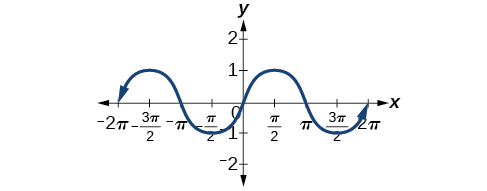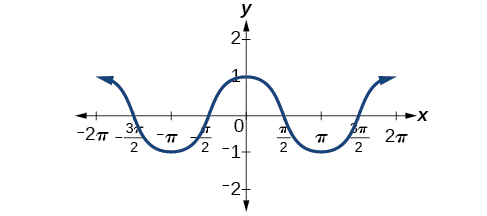6.1 Graphs of the sine and cosine functions  (Page 2/13)

 Page 2 / 13Odd symmetry of the sine function

[link] shows that the cosine function is symmetric about the y -axis. Again, we determined that the cosine function is an even function. Now we can see from the graph thatEven symmetry of the cosine function

Characteristics of sine and cosine functions

The sine and cosine functions have several distinct characteristics:

• They are periodic functions with a period of $\text{\hspace{0.17em}}2\pi .$
• The domain of each function is $\text{\hspace{0.17em}}\left(-\infty ,\infty \right)\text{\hspace{0.17em}}$ and the range is $\text{\hspace{0.17em}}\left[-1,1\right].$
• The graph of is symmetric about the origin, because it is an odd function.
• The graph of is symmetric about the $\text{\hspace{0.17em}}y\text{-}$ axis, because it is an even function.

Investigating sinusoidal functions

As we can see, sine and cosine functions have a regular period and range. If we watch ocean waves or ripples on a pond, we will see that they resemble the sine or cosine functions. However, they are not necessarily identical. Some are taller or longer than others. A function that has the same general shape as a sine or cosine function    is known as a sinusoidal function    . The general forms of sinusoidal functions are

Determining the period of sinusoidal functions

Looking at the forms of sinusoidal functions, we can see that they are transformations of the sine and cosine functions. We can use what we know about transformations to determine the period.

In the general formula, $\text{\hspace{0.17em}}B\text{\hspace{0.17em}}$ is related to the period by $\text{\hspace{0.17em}}P=\frac{2\pi }{|B|}.\text{\hspace{0.17em}}$ If $\text{\hspace{0.17em}}|B|>1,\text{\hspace{0.17em}}$ then the period is less than $\text{\hspace{0.17em}}2\pi \text{\hspace{0.17em}}$ and the function undergoes a horizontal compression, whereas if $\text{\hspace{0.17em}}|B|<1,\text{\hspace{0.17em}}$ then the period is greater than $\text{\hspace{0.17em}}2\pi \text{\hspace{0.17em}}$ and the function undergoes a horizontal stretch. For example, $\text{\hspace{0.17em}}f\left(x\right)=\mathrm{sin}\left(x\right),\text{\hspace{0.17em}}$ $B=1,\text{\hspace{0.17em}}$ so the period is $\text{\hspace{0.17em}}2\pi ,\text{}$ which we knew. If $\text{\hspace{0.17em}}f\left(x\right)=\mathrm{sin}\left(2x\right),\text{\hspace{0.17em}}$ then $\text{\hspace{0.17em}}B=2,\text{\hspace{0.17em}}$ so the period is $\text{\hspace{0.17em}}\pi \text{\hspace{0.17em}}$ and the graph is compressed. If $\text{\hspace{0.17em}}f\left(x\right)=\mathrm{sin}\left(\frac{x}{2}\right),\text{\hspace{0.17em}}$ then $\text{\hspace{0.17em}}B=\frac{1}{2},\text{\hspace{0.17em}}$ so the period is $\text{\hspace{0.17em}}4\pi \text{\hspace{0.17em}}$ and the graph is stretched. Notice in [link] how the period is indirectly related to $\text{\hspace{0.17em}}|B|.$

Period of sinusoidal functions

If we let $\text{\hspace{0.17em}}C=0\text{\hspace{0.17em}}$ and $\text{\hspace{0.17em}}D=0\text{\hspace{0.17em}}$ in the general form equations of the sine and cosine functions, we obtain the forms

$y=A\mathrm{sin}\left(Bx\right)$
$y=A\mathrm{cos}\left(Bx\right)$

The period is $\text{\hspace{0.17em}}\frac{2\pi }{|B|}.$

Identifying the period of a sine or cosine function

Determine the period of the function $\text{\hspace{0.17em}}f\left(x\right)=\mathrm{sin}\left(\frac{\pi }{6}x\right).$

Let’s begin by comparing the equation to the general form $\text{\hspace{0.17em}}y=A\mathrm{sin}\left(Bx\right).$

In the given equation, $\text{\hspace{0.17em}}B=\frac{\pi }{6},\text{\hspace{0.17em}}$ so the period will be

Determine the period of the function $\text{\hspace{0.17em}}g\left(x\right)=\mathrm{cos}\left(\frac{x}{3}\right).$

$\text{\hspace{0.17em}}6\pi \text{\hspace{0.17em}}$

Determining amplitude

Returning to the general formula for a sinusoidal function, we have analyzed how the variable $\text{\hspace{0.17em}}B\text{\hspace{0.17em}}$ relates to the period. Now let’s turn to the variable $\text{\hspace{0.17em}}A\text{\hspace{0.17em}}$ so we can analyze how it is related to the amplitude , or greatest distance from rest. $\text{\hspace{0.17em}}A\text{\hspace{0.17em}}$ represents the vertical stretch factor, and its absolute value $\text{\hspace{0.17em}}|A|\text{\hspace{0.17em}}$ is the amplitude. The local maxima will be a distance $\text{\hspace{0.17em}}|A|\text{\hspace{0.17em}}$ above the vertical midline of the graph, which is the line $\text{\hspace{0.17em}}x=D;\text{\hspace{0.17em}}$ because $\text{\hspace{0.17em}}D=0\text{\hspace{0.17em}}$ in this case, the midline is the x -axis. The local minima will be the same distance below the midline. If $\text{\hspace{0.17em}}|A|>1,\text{\hspace{0.17em}}$ the function is stretched. For example, the amplitude of $\text{\hspace{0.17em}}f\left(x\right)=4\text{\hspace{0.17em}}\mathrm{sin}\text{\hspace{0.17em}}x\text{\hspace{0.17em}}$ is twice the amplitude of $\text{\hspace{0.17em}}f\left(x\right)=2\text{\hspace{0.17em}}\mathrm{sin}\text{\hspace{0.17em}}x.\text{\hspace{0.17em}}$ If $\text{\hspace{0.17em}}|A|<1,\text{\hspace{0.17em}}$ the function is compressed. [link] compares several sine functions with different amplitudes.

find the equation of the line if m=3, and b=-2
graph the following linear equation using intercepts method. 2x+y=4
Ashley
"7"has an open circle and "10"has a filled in circle who can I have a set builder notation
x=-b+_Гb2-(4ac) ______________ 2a
I've run into this: x = r*cos(angle1 + angle2) Which expands to: x = r(cos(angle1)*cos(angle2) - sin(angle1)*sin(angle2)) The r value confuses me here, because distributing it makes: (r*cos(angle2))(cos(angle1) - (r*sin(angle2))(sin(angle1)) How does this make sense? Why does the r distribute once
so good
abdikarin
this is an identity when 2 adding two angles within a cosine. it's called the cosine sum formula. there is also a different formula when cosine has an angle minus another angle it's called the sum and difference formulas and they are under any list of trig identities
strategies to form the general term
carlmark
How can you tell what type of parent function a graph is ?
generally by how the graph looks and understanding what the base parent functions look like and perform on a graph
William
if you have a graphed line, you can have an idea by how the directions of the line turns, i.e. negative, positive, zero
William
y=x will obviously be a straight line with a zero slope
William
y=x^2 will have a parabolic line opening to positive infinity on both sides of the y axis vice versa with y=-x^2 you'll have both ends of the parabolic line pointing downward heading to negative infinity on both sides of the y axis
William
y=x will be a straight line, but it will have a slope of one. Remember, if y=1 then x=1, so for every unit you rise you move over positively one unit. To get a straight line with a slope of 0, set y=1 or any integer.
Aaron
yes, correction on my end, I meant slope of 1 instead of slope of 0
William
what is f(x)=
I don't understand
Joe
Typically a function 'f' will take 'x' as input, and produce 'y' as output. As 'f(x)=y'. According to Google, "The range of a function is the complete set of all possible resulting values of the dependent variable (y, usually), after we have substituted the domain."
Thomas
Sorry, I don't know where the "Â"s came from. They shouldn't be there. Just ignore them. :-)
Thomas
Darius
Thanks.
Thomas
Â
Thomas
It is the Â that should not be there. It doesn't seem to show if encloses in quotation marks. "Â" or 'Â' ... Â
Thomas
Now it shows, go figure?
Thomas
what is this?
i do not understand anything
unknown
lol...it gets better
Darius
I've been struggling so much through all of this. my final is in four weeks 😭
Tiffany
this book is an excellent resource! have you guys ever looked at the online tutoring? there's one that is called "That Tutor Guy" and he goes over a lot of the concepts
Darius
thank you I have heard of him. I should check him out.
Tiffany
is there any question in particular?
Joe
I have always struggled with math. I get lost really easy, if you have any advice for that, it would help tremendously.
Tiffany
Sure, are you in high school or college?
Darius
Hi, apologies for the delayed response. I'm in college.
Tiffany
how to solve polynomial using a calculator
So a horizontal compression by factor of 1/2 is the same as a horizontal stretch by a factor of 2, right?
The center is at (3,4) a focus is at (3,-1), and the lenght of the major axis is 26
The center is at (3,4) a focus is at (3,-1) and the lenght of the major axis is 26 what will be the answer?
Rima
I done know
Joe
What kind of answer is that😑?
Rima
I had just woken up when i got this message
Joe
Can you please help me. Tomorrow is the deadline of my assignment then I don't know how to solve that
Rima
i have a question.
Abdul
how do you find the real and complex roots of a polynomial?
Abdul
@abdul with delta maybe which is b(square)-4ac=result then the 1st root -b-radical delta over 2a and the 2nd root -b+radical delta over 2a. I am not sure if this was your question but check it up
Nare
This is the actual question: Find all roots(real and complex) of the polynomial f(x)=6x^3 + x^2 - 4x + 1
Abdul
@Nare please let me know if you can solve it.
Abdul
I have a question
juweeriya
hello guys I'm new here? will you happy with me
mustapha
The average annual population increase of a pack of wolves is 25.
how do you find the period of a sine graph
Period =2π if there is a coefficient (b), just divide the coefficient by 2π to get the new period
Am
if not then how would I find it from a graph
Imani
by looking at the graph, find the distance between two consecutive maximum points (the highest points of the wave). so if the top of one wave is at point A (1,2) and the next top of the wave is at point B (6,2), then the period is 5, the difference of the x-coordinates.
Am
you could also do it with two consecutive minimum points or x-intercepts
Am
I will try that thank u
Imani
Case of Equilateral Hyperbola
ok
Zander
ok
Shella
f(x)=4x+2, find f(3)
Benetta
f(3)=4(3)+2 f(3)=14
lamoussa
14
Vedant
pre calc teacher: "Plug in Plug in...smell's good" f(x)=14
Devante
8x=40
Chris
Explain why log a x is not defined for a < 0
the sum of any two linear polynomial is whatByByByBy Prateek AshtikarByBy